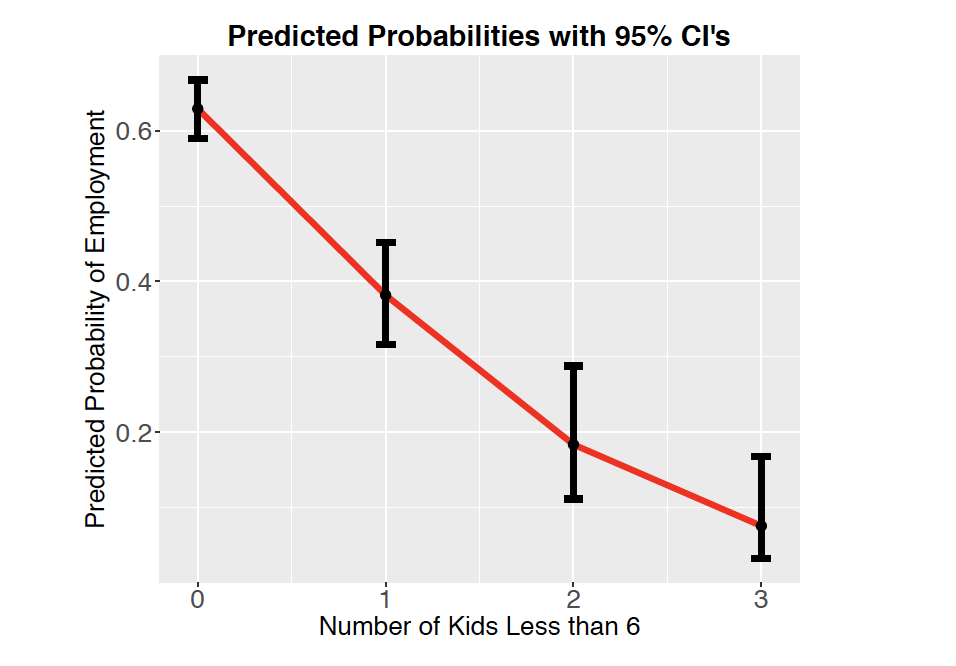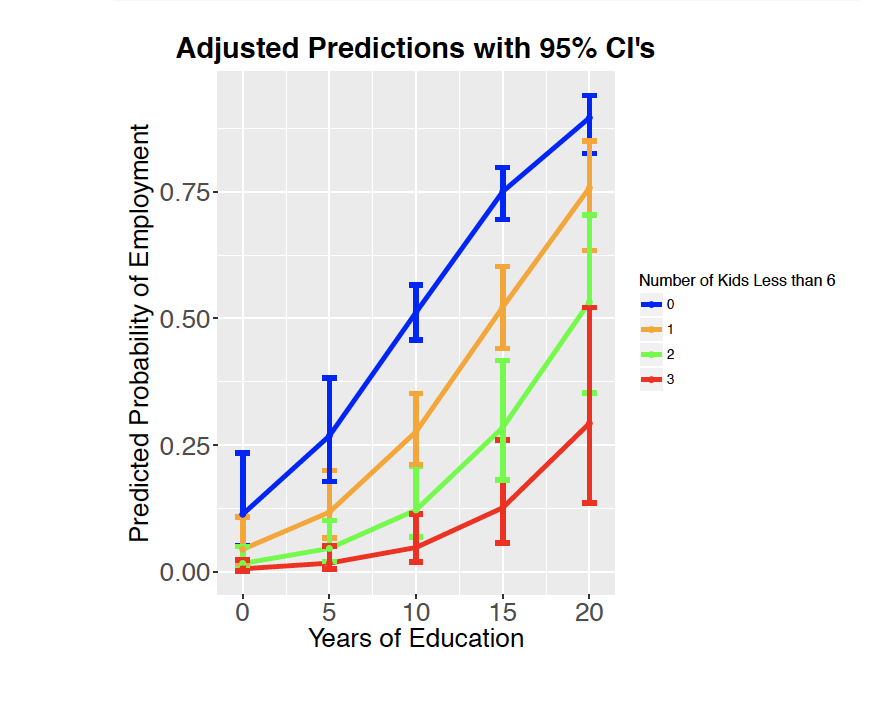# Logistic Regression Plots in R

Logistic Regression prediction plots can be a nice way to visualize and help you explain the results of a logistic regression.

Suppose we are investigating the relationship between number of kids less than 6 (the explanatory variable) and whether or not the participant is in the workforce (the response variable). A logistic regression can be used to model this relationship.

Typically, we would run a logistic regression and be able to make a conclusion such as: For each additional child under 6, it is expected that the odds of being in the workforce changes by a factor 0f 0.36. But it would be hard for this to have a tangible meaning to a non-technical audience. It is much easier to be able to SHOW them what that means with a plot!What this allows us to see is how the probability of being in the labor force is expected to decrease with each additional child and how much uncertainty we have on those estimates. For instance, it is shown that 63% of people with no kids less than 6 are expected to be employed, but we have some uncertainty on that estimate. We expect that the true proportion of people with no kids less than 6 is actually somewhere in the interval 59% to 67%. We can also see how someone with 3 kids less than 6 is expected to have about an 8% likelihood of being employed. These types of statements are usually much easier to communicate than statements about odds ratios.

It is possible to show the findings of two explanatory variables as well. This might look something like:Can you make sense of what this plot is trying to show?

You will want to start with a simple model that includes only a single explanatory variable.

## Visualizing a single explanatory variable

mod1<-glm(BinaryResponse~Explanatory, family=”binomial”, data=mydata)
summary(mod1)

#Now suppose I want to use my model to make predictions of employment likelihood

graphdata<-expand.grid(Explanatory1=c(XXXXXXX)) #Fill in with interesting values of Explanatory1

graphdata<-cbind(graphdata, PredictedProb=plogis(graphdata\$fit),
LL=plogis(graphdata\$fit-1.96*graphdata\$se.fit),
UL=plogis(graphdata\$fit+1.96*graphdata\$se.fit))

#Plot these predictions with their uncertainties
ggplot(data=graphdata)+
geom_line(aes(x=Explanatory1, y=PredictedProb), color=”red”, size=2)+
geom_errorbar(aes(x=Explanatory1, y=PredictedProb, ymin=LL, ymax=UL),width=0.1, size=2)+
geom_point(aes(x=Explanatory1, y=PredictedProb), color=”black”, size=3)

## Now suppose I have two explanatory variables in my model

mod2<-glm(BinaryResponse~Explanatory1+Explanatory2, family=”binomial”, data=mydata)
summary(mod2)

#Now suppose I want to use my model to make predictions of employment likelihood

graphdata2<-expand.grid(Explanatory1=c(XXXXX),
Explanatory2=c(XXXXX))

#Fill in the above with interesting values of Explanatory1 and Explanatory2